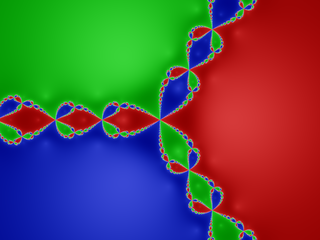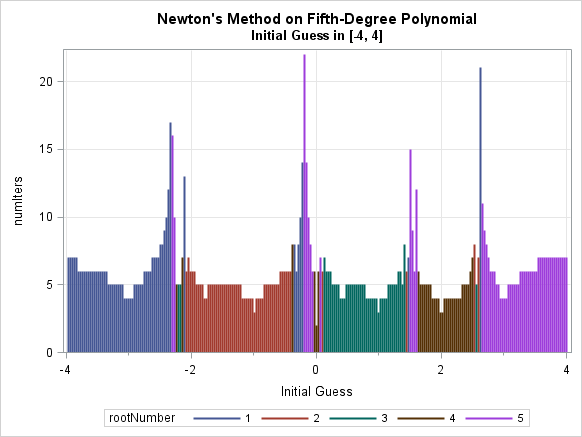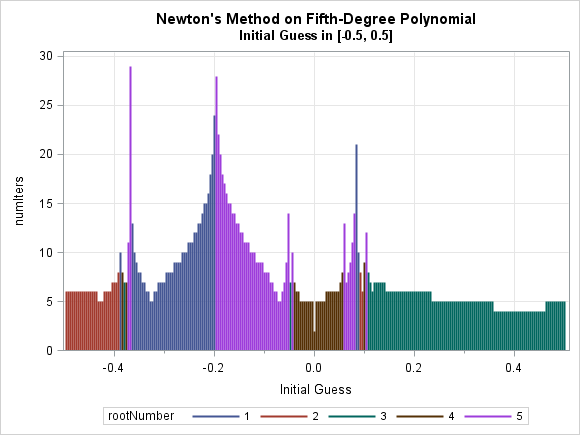In my article about finding an initial guess for root-finding algorithms, I stated that Newton's root-finding method "might not converge or might converge to a root that is far away from the root that you wanted to find." A reader wanted more information about that statement.

I have previously shown how to implement Newton's method in SAS. The behavior of Newton's method depends on the initial guess. If you provide a guess that is sufficiently close to a simple root, Newton's method will converge quadratically to the nearby root. However, if your guess is near a critical point of the function, Newton's method will produce a "next guess" that is far away from the initial guess. Further iterations might converge to an arbitrary root, might endlessly cycle in a periodic or aperiodic manner, or might diverge to infinity.

The dynamics of Newton iteration can be quite complex. If you owned a PC in the 80's and early 90's, you might have spent countless hours computing Mandelbrot sets and Julia sets. You might have seen pictures like the one at the beginning of this article, which show the domains of attraction for Newton's iteration for a cubic polynomial. In the picture, each point in the complex plane is colored according to which root Newton's method converges to when it begins at that point. The points that eventually converge to a root are the Fatou set, whereas the points that do not converge form the Julia set.

### The sensitivity of Newton's method

You can perform the same kind of computer experiments for Newton's method applied to a real function. (You can download the SAS/IML programs that I used to create the graphs in this article.) Consider the polynomial f(x) = x5 – 2 x4 – 10 x3 + 20 x2 + 9 x – 18. The roots of the polynomial are {-3, -1, 1, 2, 3}. The polynomial has critical points (where the derivative vanishes) near -2.3, -0.2, 1.5, and 2.6. Recall that Newton's method involves iteration of the rational function N(x) = xf(x)/f'(x), which has singularities at the critical points of f.

You can ask the following question: For each point, x, to which root does Newton's method converge when x is the initial guess? You can also keep track of how many iterations it takes for Newton's method to converge to a root.If you apply Newton's method to 250 initial conditions on the interval [-4, 4], you get the results that are summarized in the needle plot to the left. (Click to enlarge.) The color of the needle at x indicates the root to which x converges under Newton's method. The height of the needle indicates the number of iterations required to converge.

You can see that initial guesses that are close to a root converge to the nearby root in five or fewer iterations. Near the critical points, Newton's method requires more iterations to converge, often more than 10 and sometimes more than 20 iterations. The regions near the critical points are interlaced bands of color, which indicates that the dynamics of Newton's method is complicated in those regions. A small change in the initial guess can result in a big difference in the Newton iterations.The dynamics near 0 seem interesting, so let's zoom into that region. In particular, repeat the previous computation for 250 initial conditions on the interval [-0.5, 0.5]. The needle plot to the left reveals additional details. All roots can be reached from initial guesses in this region, even though the nearest roots are at x = -1 and x = 1 (roots #2 and #3). Again, there are regions for which many iterations are required and there is an interlacing of colors, which indicates that newton's method is sensitive to the initial guess.

You can zoom again into a multicolored region and repeat the computations on a new interval. The behavior continues ad infinitum. You can find two initial guesses that differ by an arbitrarily small amount, yet their iteration under Newton's method eventually diverge and result in different roots. This is known as sensitive dependence on initial conditions, or, more poetically, as the butterfly effect.

### Conclusions

Newton's method is one of my favorite root-finding techniques. However, it is important to understand that the famous quadratic convergence of Newton's method applies to initial guesses that are close to a root. For an arbitrary initial guess, Newton's method can be result in divergence, periodic orbits, or convergence to a far-away root. Fractals and chaos are fun topics to explore, but not when you need to find a root as part of your work. Therefore I recommend using a pre-search method, as described in my previous article, to obtain a good initial guess.

### Further details

ShareDistinguished Researcher in Computational Statistics

Rick Wicklin, PhD, is a distinguished researcher in computational statistics at SAS and is a principal developer of SAS/IML software. His areas of expertise include computational statistics, simulation, statistical graphics, and modern methods in statistical data analysis. Rick is author of the books Statistical Programming with SAS/IML Software and Simulating Data with SAS.

1.